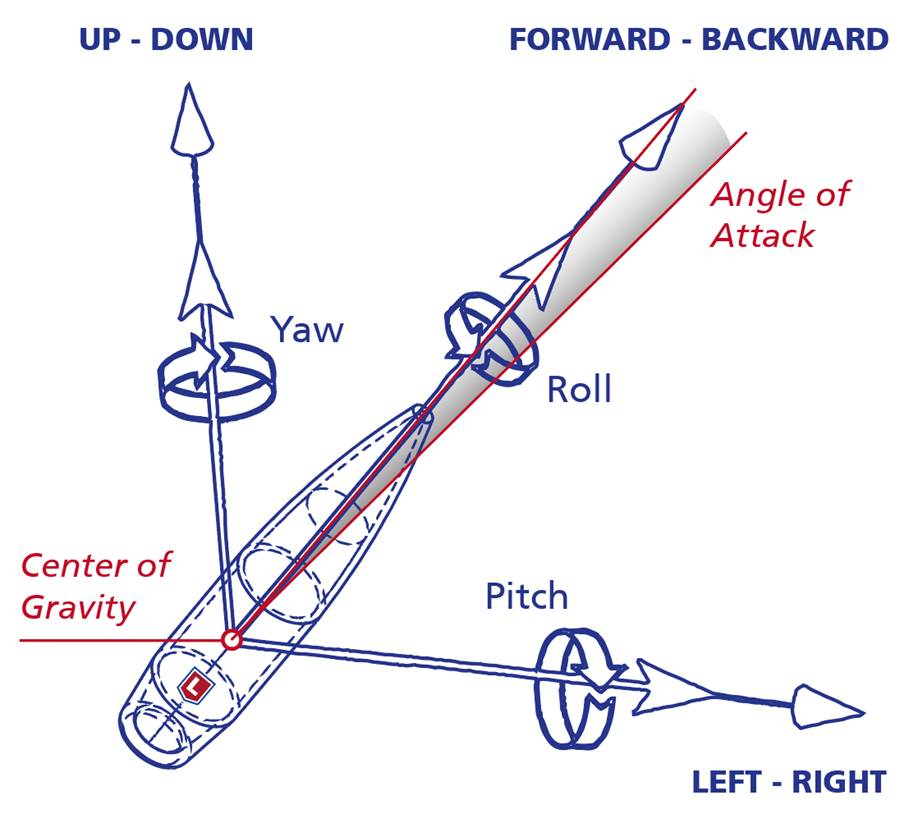# Lapua Ballistics tips: ﻿The benefits of 6DOF

## Lapua Ballistics tips: The benefits of 6DOF

(For the previous Lapua Ballistics tips go to the Lapua Ballistics page)

As most Lapua Ballistics users already know, Lapua Ballistics applies the 6DOF calculation to model flight paths for Lapua projectiles. 6DOF is also the calculation model used by Lapua Product Development. So what are the advantages of 6DOF, and what does it mean for a shooter requiring accuracy?

First, let’s take a look at some other means of modelling flight paths:

The 3DOF point-mass solution

The 3DOF calculation model considers only the drag coefficient, i.e. the Cd factor. Using 3DOF doesn’t require a large computing capacity. As the name implies, there are three degrees of freedom, i.e. translational components tracked in the equation: moving forward/backward; moving left/right; and moving up/down. 3DOF calculation doesn’t take any stance on the attitude (the orientation of the projectile relative to the direction of motion) or stability of the bullet, and has a very straight-forward and simple approach to crosswind. This means that the 3DOF model calculates the position of the bullet as a function of time reasonably correctly as long as the flight is stable. In windy or changing conditions, however, this model is not the most reliable.

The modified point-mass solution

The modified point-mass calculation (sometimes referred to as 4DOF) is more advanced than the 3D model, as the calculation includes three translational degrees of freedom and one rotational degree of freedom (spinning). The aerodynamic model includes also e.g. the contribution of lift force. These are some of the same forces that the 6DOF model calculates, however the point-mass model applies a simplified calculation which does not take into account the actual attitude of the bullet, but merely includes an algebraic approximation for the attitude (pitch/yaw angles) of the projectile. Due to some simplifications involved the model is not able to give information concerning the flight stability.

Why 6DOF?

The above mentioned calculation solutions are obviously usable for trajectory calculation. So the question is, what does the Lapua Ballistics 6DOF solution offer?

The easy answer is: the most accurate results. As with the modified point-mass model, the 6DOF calculation includes three translational degrees of freedom and three rotational degrees of freedom which give the position and attitude as a function of time. Simplified, 6DOF tracks both the pitch, yaw and roll as well as the up/down, left/right and forwards/backwards movement of the bullet.The big difference to the other calculation models is that the 6DOF models the actual trajectory path of the projectile at all times during flight, instead of just calculating points on the trajectory curve. The stability properties of the bullet are obtained during the trajectory integration since all the rotational degrees of freedom are present in simulations. Also, the stability analysis of the Lapua ballistics app is more advanced than most other calculators, as it considers both dynamic (Sd) and gyroscopic (Sg) stability factors, not only the latter as many other ballistic calculators do. The Sd is especially important in long range shooting for analyzing the transonic stability of the bullet.

But how can the Lapua Ballistics app utilize 6DOF? It is after all a very complicated mass of data, something a mobile phone would not be able to calculate.

The explanation lies in the background information of the app data. Every 6DOF-calculation is based on an aerodynamic values table that is calculated for every Lapua bullet. The table includes several aerodynamic coefficients and bullet specifics: Drag coefficient derived from Doppler radar data, Normal force coefficient slope, pitching moment coefficient slope, roll damping moment coefficient, two stability derivate, Magnus force coefficient slope and Magnus force moment. All those coefficients must be functions of the Mach number.

These calculated values are taken into account for every Lapua bullet in the Lapua Ballistics app. This is also why we can’t provide the same level of accuracy for other bullets; utilizing 6DOF requires this background data.

Accuracy comes down to not only data, but to weather, equipment, mental preparedness and so on.  The Lapua Ballistics app gives you the best data to take that perfect shot. The rest is up to you.